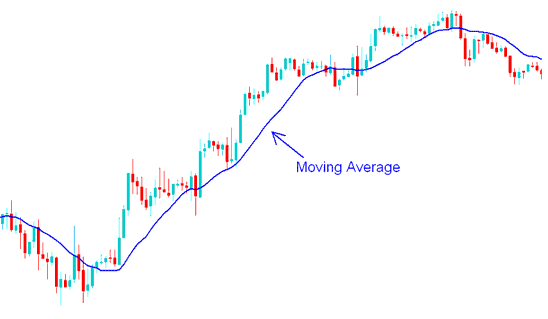# About the Moving Average Forex Strategy

Forex Moving average is one of the most widely used Forex Trading Indicator because it is simple and easy to use.

This Forex Indicator is a trend following indicator that is used by Forex traders for three things:

• Identify the beginning of a new forex market trend

• Measure the sustainability of the new forex trend

• Identify the end of a forex trend and signal a reversal forex trading signal

The Forex Moving Average or Forex MA is used to smooth out the volatility of forex price action. The MA is an overlay forex technical indicator and it is placed on top or superimposed on the forex price chart.

On the example forex trading chart below the blue line represents a 15 period MA, which acts to smooth out the volatility of the forex price action.Forex Moving Average Technical Indicator - MetaTrader 4 Forex Chart Indicators

## Calculation of the Moving Average

The Forex Moving Average is also known as MA - is calculated as an average of forex price using the most recent price data.

If the MA uses the 10 period to calculate the average of the forex price then it is referred to as a 10 period forex moving average, because most forex traders use the day as the standard price period we shall just refer to it as the 10 day MA.

To calculate the ten day MA the price of the last 10 days is averaged, the forex moving average average indicator is then updated constantly after every new forex price period. So after every new forex price period is formed the moving average is then calculated afresh using the most recent 10 forex price periods, that is why it is called a moving average because the average is constantly moving when forex price data is updated.